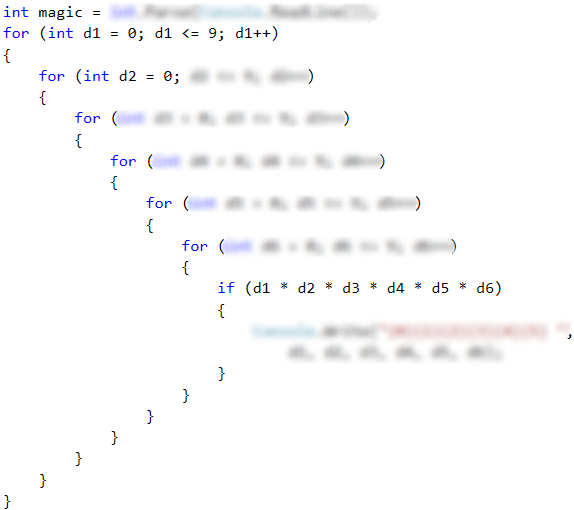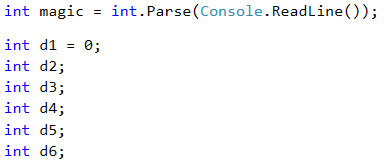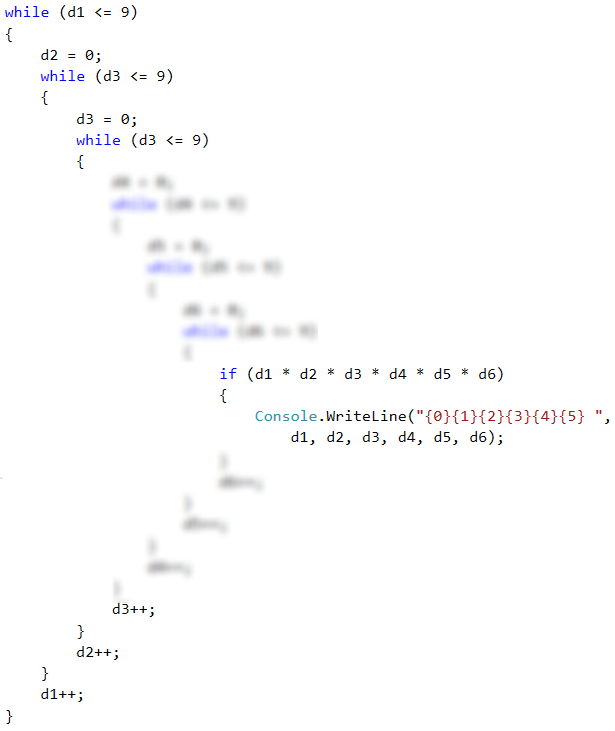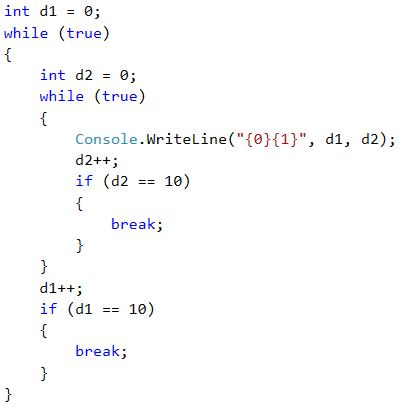# Problem: Magic Numbers

Write a program that enters a single integer magic number and produces all possible 6-digit numbers for which the product of their digits is equal to the magical number.

Example: "Magic number" → 2

• 111112 → 1 * 1 * 1 * 1 * 1 * 2 = 2
• 111121 → 1 * 1 * 1 * 1 * 2 * 1 = 2
• 111211 → 1 * 1 * 1 * 2 * 1 * 1 = 2
• 112111 → 1 * 1 * 2 * 1 * 1 * 1 = 2
• 121111 → 1 * 2 * 1 * 1 * 1 * 1 = 2
• 211111 → 2 * 1 * 1 * 1 * 1 * 1 = 2

## Input Data

The input is read from the console and consists of one integer within the range [1 … 600 000].

## Output Data

Print on the console all magic numbers, separated by space.

## Sample Input and Output

Input Output Input Output Input Output
2 111112 111121 111211 112111 121111 211111 8 111118 111124 111142 111181 111214 111222 111241 111412 111421 111811 112114 112122 112141 112212 112221 112411 114112 114121 114211 118111 121114 121122 121141 121212 121221 121411 122112 122121 122211 124111 141112 141121 141211 142111 181111 211114 211122 211141 211212 211221 211411 212112 212121 212211 214111 221112 221121 221211 222111 241111 411112 411121 411211 412111 421111 811111 531441 999999

## Solution using a "Fr" Loop

The solution follows the same concept (again we need to generate all combinations for the n element). Following these steps, try to solve the problem yourself.

• Declare and initialize variable of int type and read the input from the console.
• Nest six for loops one into another, for every single digit of the searched 6-digit numbers.
• In the last loop, using if construction, check if the product of the six digits is equal to the magic number.## Solution using a "While" Loop

In the previous chapter we reviewed other loop constructions. Let's look at the sample solution of the same problem using the while loop.

First, we need to store the input magical number in a suitable variable. Then we will initialize 6 variables – one for each of the six digits of the searched numbers.### Writing a While Loop

Then we will start writing while loops.

• We will initialize first digit: d1 = 0.
• We will set a condition for each loop: the digit will be less than or equal to 9.
• In the beginning of each loop we set a value of the next digit, in this case: d2 = 0. In the nested for loops we initialize the variables in the inner loops at each increment of the outer ones. We want to do the same here.
• At the end of each loop we will increase the digit by one: d++.
• In the innermost loop we will make the check and if necessary, we will print on the console.### Infinite While Loop

Let's remove the if check from the innermost loop. Now, let's initialize each variable outside of the loops and delete the rows dx = 0. After we run the program, we only get 10 results. Why? What if you use do-while? In this case this loop does not look appropriate, does it?Think why. Of course, you can solve the problem using an infinite loop.As we can see, we can solve a problem using different types of loops. Of course, each task has its most appropriate choice. In order to practice each type of loops – try to solve each of the following problems with all the learned loops.